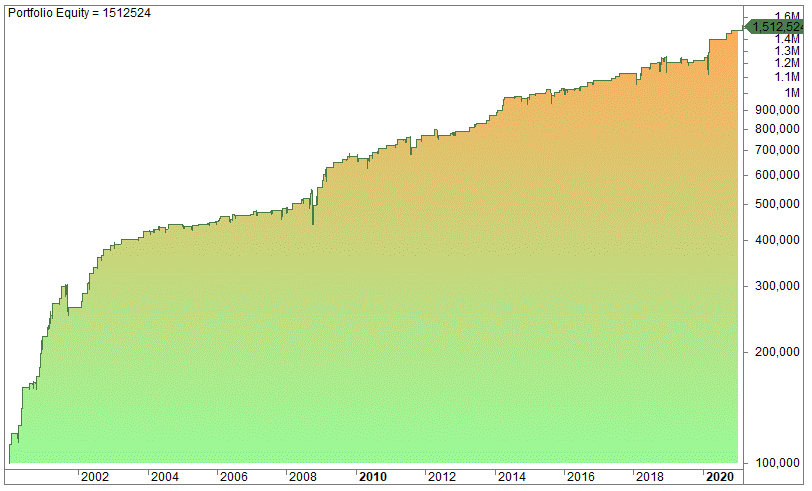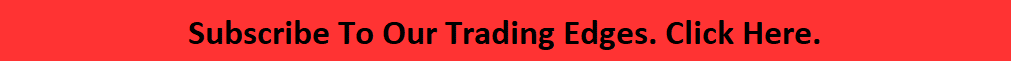Average True Range Trading Strategy (ATR Indicator – How To Use It))

Last Updated on December 16, 2021 by Oddmund Groette

The ATR indicator is useful on its own and can be used to develop an Average True Range trading strategy. The Average True Range (ATR) was developed by Welles Wilder in the 1970s. In his famous book called New Concepts in Technical Trading Systems, published in 1978, Wilder also published the RSI and the ADX indicators.

In this article, we look at the Average True Range (ATR) indicator. We explain what the Average True Range indicator is, how it works, how you can use it, and if it’s possible to make profitable strategies by using the ATR. We show you why ATR is a useful indicator.

Welles Wilder was ahead of his time, this was well before the computer age, and all his indicators have stood the test of time and are still widely used. To this day, the RSI is probably the most popular trading indicator on the planet.

What is the Average True Range (ATR indicator)?

The Average True Range is a mathematical indicator that sets to measure the range of price changes. The ATR is calculated in two steps – the true range and the average of the true range:

How to calculate the True Range:

The true range is the greatest of these three calculations:

• High minus the previous close
• High minus low
• The previous close minus low

How to calculate the Average True Range:

When we know the true range of each day/bar, the range is calculated by using x-day/bar of the maximum range every day.

In this article, we use the in-built ATR in Amibroker, which is based on the original formula by Wilder in the book mentioned above. Wilder used a smoothed moving average that was built upon an exponential moving average. Read more here for the difference between simple and moving averages:

The Average True Range is a volatility measure

As you might have guessed the ATR is first and foremost a volatility measure that gives you no clue about the direction of the price: it could be up or it could be down. However, volatility normally picks up when the markets are uncertain.

The chart below shows the 15-day ATR in QQQ (Nasdaq):During the Covid-19 in March and April 2020, the volatility was very high but has since then cooled off.

How to use the Average True Range indicator

There are many ways to use the ATR. Below you find the four most obvious ways to use ATR:

Average True Range as a volatility filter:

The ATR is frequently used as a volatility filter. You can, for example, scan stocks to filter out both low and high volatility stocks. Some stocks perform better than others, and you might want certain stocks within a sub-sector based on volatility. Volatility is often a very good filter.

Average True Range as stop-loss:

ATR is often used to set stop-loss levels. For example, you can put a stop loss 1.5 times ATR lower than the entry price (if you are long). If your entry is 100 and the 20-day ATR is 5.5, then your trigger point for the stop-loss is 91.75 (100 minus 5.5 times 1.5). In other words, if the price drops to 91.75 you sell to cut your losses.

Average True Range as position sizing:

Another way to use ATR is to adjust position sizing. If you are trading a portfolio of ten stocks, each of them might have different volatility. It doesn’t make sense to trade the same size in stocks that might be completely different in terms of volatility.

For example, let’s say you have a portfolio of ten stocks that you trade on a rotational basis. To adjust for volatility you can take 10 000 (or whatever number you set as default) and divide by the Average True Range over the last 100 days. This way you allocate more capital to the least volatile stocks compared to the ones more volatile.

Average True Range can be used to build trading strategies:

Furthermore, you can build trading strategies based on the ATR, mostly mean-revertive strategies. However, most of these strategies are often overlapping trades with the RSI  (for example). Below you find some examples of how to develop such strategies:

How to use Average True Range in the S&P 500

Average True Range can, of course, be used on any financial asset there is, even crypto. However, the most traded asset in the US is indices for stocks, and below we have provided some examples of very simple strategies. They are profitable, but they need to be worked upon to be used as stand-alone strategies.

Let’s first test using a very simple hypothesis:

Buy when the close is below a moving average deducted from the Average True Range, and sell when the opposite is true. The code in Amibroker is like this:

Buy = C < MA(C,10) – (ATR(10)*1.5);
Sell = C>MA(C,10) + (ATR(10)*1.5);
sellPrice = Close ;

In plain English it reads like this:

Buy when the close is below the ten-day moving average of the closing price deducted the ten-day Average True Range multiplied with 1.5. Sell when the opposite is true.

Both the entry and the exit are done at close.

On the S&P 500, we get this equity curve:This is, to say the least, not tradeable. What happens if we lower the exit criteria? Let’s exit when the close is higher than the 10-day moving average:

Buy = C < MA(C,10) – (ATR(10)*1.5);
Sell = C>MA(C,10);
sellPrice = Close ;

The equity curve improves, but it’s still not tradeable:What happens if we perform the last strategy on Nasdaq? On the ETF QQQ we get this equity curve:It’s better, but still not acceptable.

Nevertheless, the strategy can still be improved. We added two more variables to the buy criteria and changed the exit. The strategy improves a lot, both on Nasdaq and the S&P 500. This is the new equity chart for Nasdaq:Even if we ignore the best trades prior to 2004, the result is pretty good: the profit factor is 3.1.

If we use the same system on the S&P 500 the equity curve improves as well:Both of the above equity charts are based on swing trading where the holding period is a few days.

The last strategy above produces this equity curve if we exit on the next day open:If you want to have the code for the strategy producing the three last equity charts (including Amibroker and Tradestation code plus “plain English”), you can order it for 75 USD via this link (ATR Strategy no. 1):When you have paid, please press the link below to access the code (PDF file):

Download ATR strategy no.1 by clicking here (you need to pay for access)

OR

You can subscribe to our Trading Ideas where we send out ideas like this monthly. The edge above will be presented as an edge in a few months. If you’d like to receive similar ideas, please subscribe to our Trading Edges:Conclusion: Average True Range strategy

Average True Range can be used as a trading tool in many ways. It’s a versatile instrument that can be used for position sizing, finding stop-loss levels, volatility filters, and building trading strategies. It’s such a useful indicator that it can be used on its own to develop an Average True Range strategy.